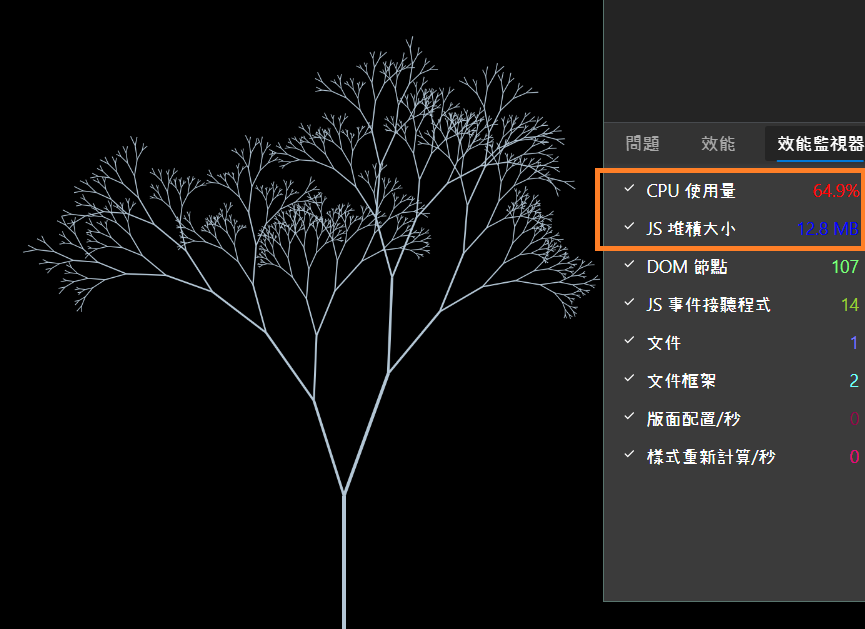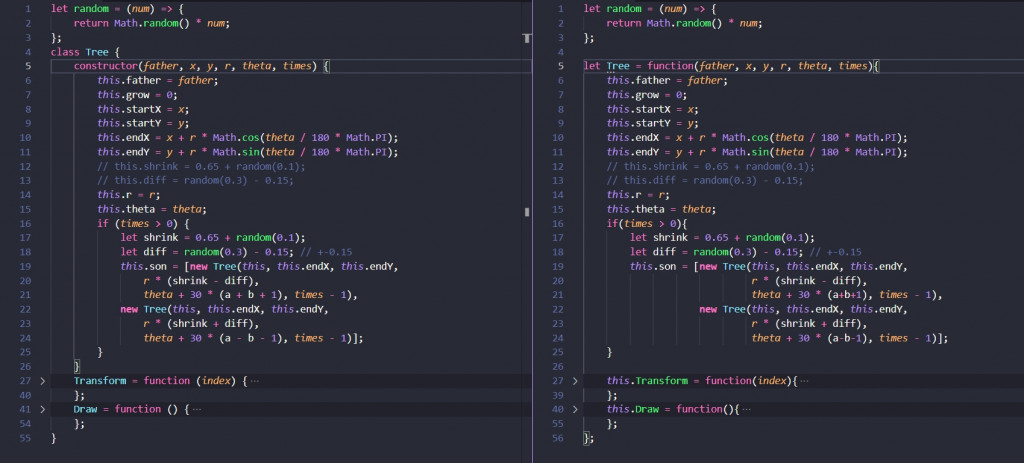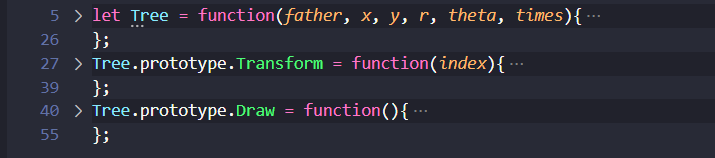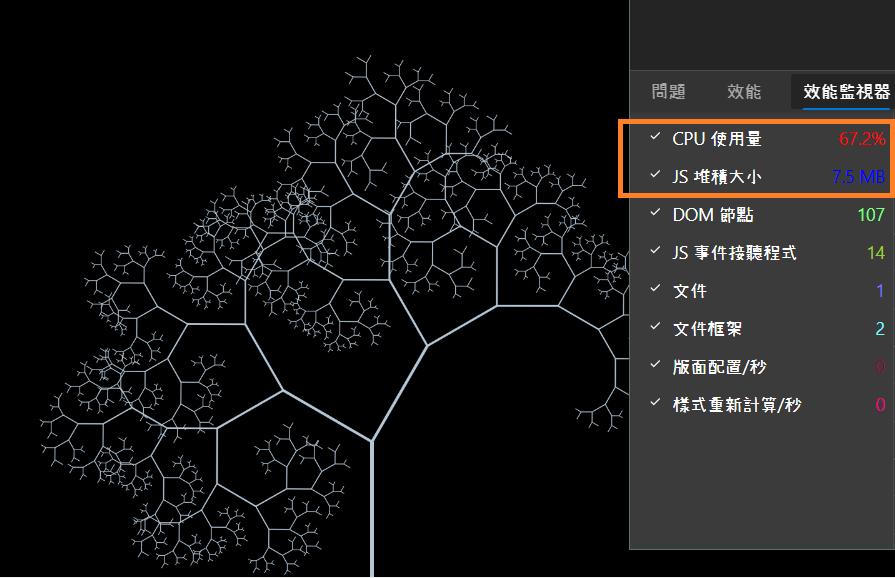#DAY 17
0
Modern Web

## 先補上Demo

https://jerry-the-potato.github.io/Chapter3-demo-object/### 試試看ClassesClass把原本的建構式拆出來寫，結構上可以較清楚在實例化的當下會建構哪些變數

### 回到物件## 來處理樹枝的細節

### 幫樹枝添加層次

``````let alpha = 0.5 + 0.5 * (r / (window.innerHeight/3));
context.strokeStyle = 'rgba(179, 198, 213, ' + alpha + ')';
``````

### 讓樹慢慢長出來

``````let x = (this.startX - WIDTH / 2) * this.grow + WIDTH / 2,
y = (this.startY - HEIGHT) * this.grow + HEIGHT,
r = this.r * this.grow,
this.grow = Math.min(this.grow + 0.01 / (0.8 + 2 * this.grow), 1);
``````

### 以上完整程式碼

``````Tree.prototype.Draw = function () {
let x = (this.startX - WIDTH / 2) * this.grow + WIDTH / 2,
y = (this.startY - HEIGHT) * this.grow + HEIGHT,
r = this.r * this.grow,
theta = this.theta;
context.beginPath();
context.moveTo(x, y);
context.lineTo(x + r * Math.cos(theta / 180 * Math.PI),
y + r * Math.sin(theta / 180 * Math.PI));
context.lineWidth = 1 + r / 50;
let alpha = 0.5 + 0.5 * (r / (window.innerHeight/3));
context.strokeStyle = 'rgba(179, 198, 213, ' + alpha + ')';
context.stroke();
// 如果有子樹枝，就繼續呼叫所有的子樹枝
if (this.son) this.son.forEach(branch => branch.Draw()); // 遞迴
this.grow = Math.min(this.grow + 0.01 / (0.8 + 2 * this.grow), 1);
};
``````

### 1 則留言

1

https://ithelp.ithome.com.tw/articles/10272091